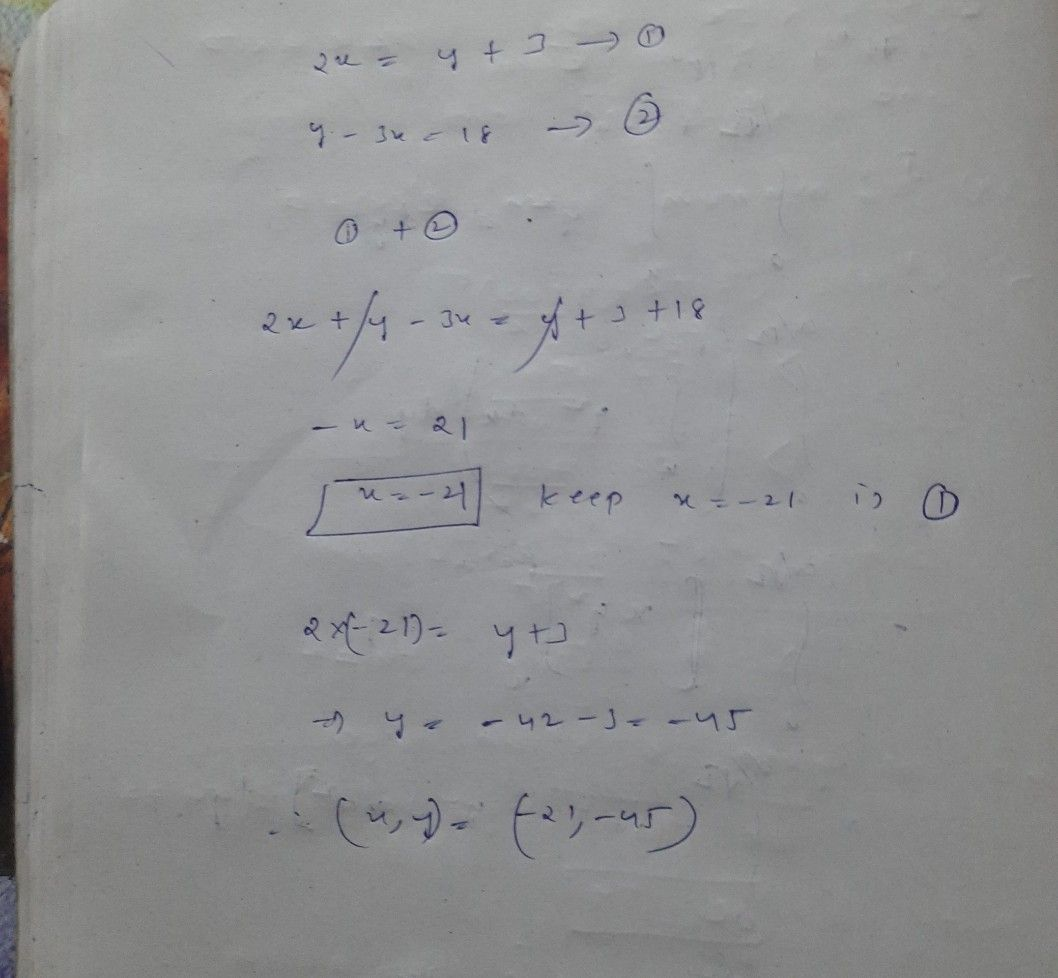Symbol
Problem$c26$ $-$ E $-y+3$ $9=3\times =18$
10th-13th grade
Other
SolutionQanda teacher - GAITONDEStudent
ty so much sirQanda teacher - GAITONDE
you are welcome